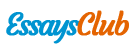# Hp Deskjet Case - Assignment 3

Autor:   •  May 24, 2018  •  1,273 Words (6 Pages)  •  265 Views

Page 1 of 6

...

- We can find the average inventory cost per printer by dividing the annual average inventory cost by mean annual demand (mean monthly demand × 12).

- The total supply chain cost per printer sold is given by (unit cost) + (average inventory cost per printer) + (transportation cost per printer).

- Your recommendation to HP should be to use the option that minimizes total inventory cost per printer.

“Current Policy” for Question 2:

The “current policy” used by HP is not clearly explained in the case. According to the case, the “the target inventory levels at the DCs were based on safety stocks that were a result of some judgmental rule of thumb” (p. 7), which you should assume is equal to one-month’s average sales. To be consistent, use the following interpretation: “Target physical inventory level at the DC” = “Average physical inventory at the DC”. Recall that

(avg physical inv) = (avg cycle stock) + (safety stock),

whereas

(avg inv position) = (avg physical inv) + (avg pipeline inv).

Thus if we are keeping one month’s average sales as physical inventory, then we have

(1 month’s avg sales) = (avg cycle stock) + (implied level of SS), or

SS = (1 month’s avg sales) – (avg cycle stock).

Since we know that

(avg cycle stock) = (avg demand during T)/2,

and since T = 1 week, we get

SS = (1 month’s avg sales) – (1 week’s avg sales)/2.

Under the fixed time-period (periodic review) model, the average inventory at the DC is the cycle stock plus the safety stock (= Q/2 + SS), where the cycle stock is half of the sales during the review period. For example, for Option A one-month’s average sales = 42.3 units, i.e., one-week’s average sales = 42.3/4.33 = 9.8 units. If the average physical inventory at the DC is set to 42.3 units, then:

42.3 = (avg cycle stock) + Safety Stock

Safety Stock = 42.3 − (avg cycle stock) = 42.3 − (9.8)/2 = 37.4 items.

You should be able to compute that if the safety stock is 37.4, then the service level for Option A will be less than 98%. So, under the “current policy,” inventory for Option A will probably be lower than what you computed in Question 1 (and thus inventory cost will be lower), but the service level for Option A will be lower than the required 98%.

Note that pipeline (or in-transit) inventory is not held by the DC, but its cost should be included in the supply chain cost.

Suggested length: 2 pages, possibly more when Excel printouts are included.

...